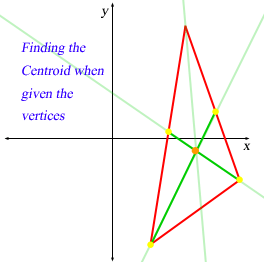SEARCH HOMEMath Central Quandaries & QueriesQuestion from Josh, a teacher: vertices X(-2,6) Y(4,10) Z(14,6) find the cooridinates of the centroidHi Josh.The centroid (see the definition in our glossary) can be found just by the intersection of two of the medians, since the third will just go through the same point.

So let M be the midpoint of X and Y: it is at location ( (-2 + 4) / 2, (6 + 10) / 2 ) = (1, 8).

Let N be the midpoint of X and Z: it is at location ( (-2 + 14) / 2, (6 + 6) / 2) = (6, 6).

So you want to find the intersection of lines YN and ZM.

Use the point-point form of a line to find the equations of the two lines, then solve for the intersection by using the substitution or elimination methods.

Cheers,
Stephen La Rocque.Math Central is supported by the University of Regina and The Pacific Institute for the Mathematical Sciences.